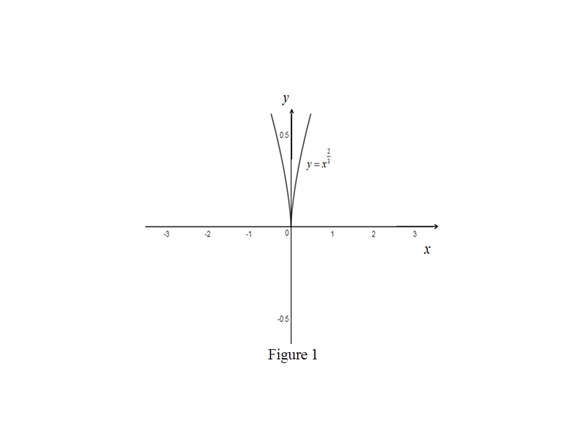# The function g ′ ( 0 ) does not exist.### Single Variable Calculus: Concepts...

4th Edition
James Stewart
Publisher: Cengage Learning
ISBN: 9781337687805### Single Variable Calculus: Concepts...

4th Edition
James Stewart
Publisher: Cengage Learning
ISBN: 9781337687805

#### Solutions

Chapter 2.7, Problem 50E

(a)

To determine

## To Show: The function g′(0) does not exist.

Expert Solution

### Explanation of Solution

Result Used: The derivative of a function at x=a is given by formula,

g(a)=limxag(x)g(a)xa (1)

Proof:

Consider the function, g(x)=x23.

Compute g(0) by using the equation (1),

g(0)=limx0g(x)g(0)x0=limx0x23(0)23x=limx0x23x=limx0xx13x

=limx01x13

Here, the function 1x13 becomes larger and larger as x tends to zero. That is,

g(0)=limx01x13=

Therefore, the derivative of the function does not exist at x=0.

Thus, the required proof is obtained.

(b)

To determine

### To find: The value of g′(a) if a≠0.

Expert Solution

The value of derivative of f(x) at x=a is 23a13.

### Explanation of Solution

Given:

The function y=x23.

Calculation:

Obtain the derivative of the function g(x) at x=a.

Compute g(a) by using the equation (1),

g(x)=limxag(x)g(a)xa=limxax23a23xa=limxa(x13)2(a13)2(x13)3(a13)3=limxa(x13+a13)(x13a13)(x13a13)(x23+a23+x13a13)

Simplify further,

g(a)=limxa(x13+a13)(x23+a23+x13a13)=((a)13+a13)((a)23+a23+(a)13a13)=2a133a23=23a13

Thus the value of the derivative at x=a is, g(a)=23a13.

(c)

To determine

Expert Solution

### Explanation of Solution

Result Used:

A curve has a vertical tangent line at x=a if g is continuous at x=a and limxa|g(x)|=

Proof:

Consider the equation y=x23.

Substitute x=0 in g(x),

g(0)=(0)23=0

Thus g(0)=0 is defined.

The limit of the function g(x) is computed as follows,

limx0g(x)=limx0x23=(0)23=0

Therefore, g(x) is continuous at x=0.

From part (b), g(x)=2x133.

Take the limit of the function g(x) as x approaches zero.

limx0|g(x)|=limx0|2x133|=23limx0|x13|=23limx0|1x13|=23limx01|x13|

=

Since the function g(x) is continuous at x=0 and limx0|g(x)|=.

By result, the curve g(x) has a vertical tangent at x=0.

Thus, the curve g(x) has a vertical tangent at the point (0,0).

(d)

To determine

Expert Solution

### Explanation of Solution

Graph:

Use the online graphing calculator to draw the graph of the function y=x23 as shown below in Figure 1,Illustration:

From Figure 1, it is clear that the y-axis is the vertical tangent to the curve y=x3.

### Have a homework question?

Subscribe to bartleby learn! Ask subject matter experts 30 homework questions each month. Plus, you’ll have access to millions of step-by-step textbook answers!